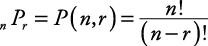## Permutations

Multiplication principle for events: If one event can occur in p different ways, and another independent event can occur in q different ways, then there are pq ways that both events can occur together.

##### Example 1

How many different ways can a man coordinate a wardrobe if he has a choice of four different pants and two different sport jackets?

The first event (selecting pants) can occur in four different ways. The second event (selecting a sport jacket) can occur in two ways. Therefore, according to the multiplication principle for events, there are (4)(2) = 8 different ways to select a wardrobe.

##### Example 2

In how many ways can five books be arranged on a shelf?

The first space can be filled with any of the five books, the second space with any of the remaining four books, the third space with any of the remaining three books, the fourth space with either of the remaining two books, and the fifth space with the last book. Therefore, there are (5)(4)(3)(2)(1) = 120 ways to arrange five books on a shelf.

##### Example 3

In how many ways can three out of eight books be arranged on a shelf?

The first space can be filled in any of eight ways, the second space in any of the remaining seven ways, the third space in any of the remaining six ways. Therefore, there are (8)(7)(6) = 336 ways that three out of eight books can be arranged on a shelf.

The arrangement of objects in a certain order is called a permutation. The number of ways to arrange eight things taken three at a time is written as P(8, 3) or as 8 P 3. P( n, r) or nP r is read as “the permutation of n things taken r at a time.”If any of the objects in a permutation are repeats, a different formula is used. The number of permutations of n objects of which p are alike and q are alike is##### Example 4

How many different ways can the letters in the word “Mississippi” be arranged?

“Mississippi” has eleven letters. Since “i” is repeated four times, “s” is repeated four times, and “p” is repeated two times, then there aredifferent ways that the letters in the word “Mississippi” can be arranged.